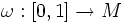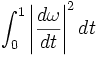# Energy functional

Fix a Riemannian manifold. The energy functional is a map from the space of piecewise smooth paths on the manifold, to reals, defined as follows. The energy functional of a curve$\omega:[0,1] \to M$ is:$\int_0^1 \left|\frac{d\omega}{dt}\right|^2 dt$
The energy functional is thus parametrization-dependent, viz if we take an increasing function$f:[0,1] \to [0,1]$ the energy functional evaluated at$\omega$ and at$\omega \circ f$ may give different values.